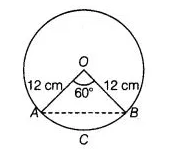# Find the area of the segment of a circle

Question:

Find the area of the segment of a circle of radius 12 cm whose corresponding sector has a centrel angle of 60°. (use π = 3.14)

Solution:

Given that, radius of a circle (r) = 12 cm

and central angle of sector OBCA (θ) = 60°$\therefore \quad$ Area of sector $O B C A=\frac{\pi r^{2}}{360} \times \theta$ [here, $O B C A=$ sector and $A B C A=$ segment ]

$=3.14 \times 2 \times 12$

$=3.14 \times 24=75.36 \mathrm{~cm}^{2}$

Since, $\triangle O A B$ is an isosceles triangle.

Let $\angle O A B=\angle O B A=\theta_{1}$

and $\quad O A=O B=12 \mathrm{~cm}$

$\angle A O B=\theta=60^{\circ}$

$\therefore$$\angle O A B+\angle O B A+\angle A O B=180^{\circ} \quad\left[\because\right.$ sum of all interior angles of a triangle is $\left.180^{\circ}\right]$\

$\Rightarrow \quad \theta_{1}+\theta_{1}+60^{\circ}=180^{\circ}$

$\Rightarrow \quad 2 \theta_{1}=120^{\circ}$

$\Rightarrow \quad \theta_{1}=60^{\circ}$

$\theta_{1}=\theta=60^{\circ}$

So, the required $\triangle A O B$ is an equilateral triangle.

Now, area of $\Delta A O B=\frac{\sqrt{3}}{4}(\text { side })^{2}$ $\left[\because\right.$ area of an equilateral triangle $\left.=\frac{\sqrt{3}}{4}(\text { side })^{2}\right]$

$=\frac{\sqrt{3}}{4}(12)^{2}$

$=\frac{\sqrt{3}}{4} \times 12 \times 12=36 \sqrt{3} \mathrm{~cm}^{2}$

Now, area of the segment of a circle i.e., $A B C A=$ Area of sector $O B C A$ - Area of $\triangle A O B$

$=(75.36-36 \sqrt{3}) \mathrm{cm}^{2}$

Hence, the required area of segment of a circle is $\left(75.36-36 \sqrt{3)} \mathrm{cm}^{2}\right.$.